# Spectrum of a commutative unital ring

## Definition

The spectrum of a commutative unital ring is a highly structured object that captures much of the geometry associated with the ring. We here describe its structure at various levels.

### Set-theoretic structure

Set-theoretically, the spectrum is the set of prime ideals in the ring.

### Topological structure

A subset in the spectrum is deemed a closed set if and only if there exists a radical ideal of the ring such that the given subset is precisely the set of primes containing that radical ideal. Since every radical ideal is the intersection of the prime ideals containing it, there is a bijective correspondence between closed subsets of the spectrum and radical ideals of the ring.

This topology is sometimes termed the Zariski topology though it should not be confused with the Zariski topology for a polynomial ring over an algebraically closed field.

The topology described above on the spectrum can be derived very naturally from the Galois correspondence between a ring and its spectrum: the closed sets in the topology are the same as the sets which are closed in the sense of the Galois correspondence.

### Structure of a locally ringed space

The spectrum can also be given the structure of a locally ringed space. The structure is as follows:

• To each open set, associate the localization at the saturated subset which is the complement of the union of prime ideals corresponding to points in the open set.
• The restriction maps of the sheaf is the natural map from the localization at a smaller saturated subset, to the localization at a larger multiplicative system
• The stalk at each point is a local ring, which is the localization at the prime ideal represented by that point

A locally ringed space that can be realized as the spectrum of a commutative unital ring in the above fashion, is termed an affine scheme.

## Functoriality

Further information: induced map on spectra by a ring homomorphism

The spectrum is a contravariant functor from the category of commutative unital rings, to the category of sets, topological spaces, or locally ringed spaces, depending on how much structure we impose.

### Set-theoretic structure

Suppose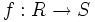$f:R \to S$ is a homomorphism of commutative unital rings. Then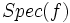$Spec(f)$, sometimes denoted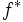$f^*$, is the map from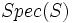$Spec(S)$ to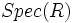$Spec(R)$ which sends a prime ideal of$S$ to its contraction in$R$. The fact that the map is well-defined follows from the fact that the contraction of a prime ideal is prime.

### Topological structure

The map$Spec(f)$ is, in fact, a continuous map with respect to the topologies on$Spec(S)$ and$Spec(R)$.

### Structure of a locally ringed space

Fill this in later

## Facts

### Topology of spectrum captures only the reduced part

The topological space structure of the spectrum ignores nilpotents, in the sense, that it depends only on the quotient of the ring by its nilradical (viz, the corresponding reduced ring).

### Topological space properties of the spectrum

The spectrum satisfies the following topological properties:

### Correspondence between ideal properties and topological properties of subsets

Under the bijective correspondence between radical ideals and closed subsets, the following property correspondence is established:

• Prime ideals correspond to the closure of one-point subsets
• Maximal ideals correspond to closed points
• Minimal prime ideals correspond to those closures of one-point subsets that are not contained in the closures of any other one-point subset. Equivalently, minimal primes correspond to maximal irreducible closed subsets. For a Noetherian ring, the minimal primes are precisely the irreducible components of the spectrum

### Correspondence between ring properties and properties of the spectrum

The converse in each case holds if we further assume that the ring is a reduced ring:

• The spectrum of a Noetherian ring is a Noetherian space
• The spectrum of an integral domain is one where there is a dense point (the point corresponding to the zero ideal). Equivalently, it is an irreducible space
• The spectrum of a field is a single point
• The spectrum of a zero-dimensional ring is a$T_1$ space
• The spectrum of a Jacobson ring is one where every closed set is the closure of the subset of its closed points

### Correspondence between ring operations and spectra

• The spectrum is a contravariant functor, as mentioned earlier
• The spectrum of the direct limit of a directed system of commutative unital rings, is the inverse limit of their spectra
• The spectrum of a direct product of two commutative unital rings, is a disjoint union of the spectra